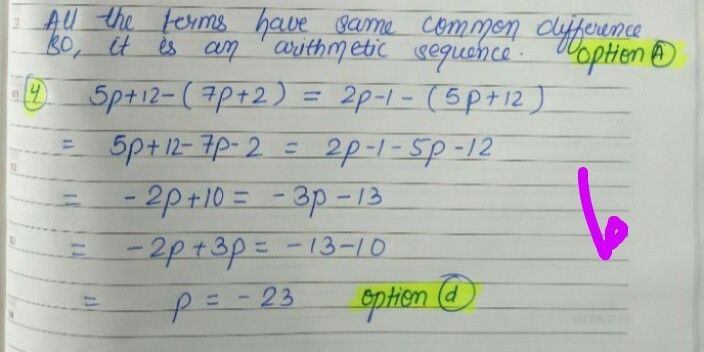Symbol
Problem$1$ $on$ Read each item carefully. Encircie the letter that coresponds to your answer. 1. Find the eight term of a geometric sequence where the third tern is $27$ and the common ratio is 3. $A$ $2187$ B. $6561$ C. $19683$ $0.59049$ $2$ Find $ps0$ that the numbers $7p+2,5p+12,2p-1,...$ $fom$ an arithmetic sequence. $A$ $-5$ $B-8$ $C$ $13$ $D$ $-23$
10th-13th grade
Other
Search count: 106
SolutionQanda teacher - missPreetiIf you have any doubts please do let me know dear. if you are satisfied with my answer please do LIKE and give a 5 STAR RATING and coins as THANK U GIFT..! ALL THE BEST..!!!If you have more questions then please ask through preffered match from my profileStudent
THANKYOU MA'AMQanda teacher - missPreeti
To evaluate:- 1. Click on top right corner evaluate button. 2. Select 5 star 3. Click on gift coins + three times. 4. Click on DONE button on top right corner.